The cathode resistor, used for self-bias, introduces a form of local negative feedback: when the current increases, the cathode voltage increases as well reducing the grid to cathode voltage, and vice versa. The result is that the gain of the voltage amplifier with self-bias is reduced. This local negative feedback can be significantly neutralised by using a bypass capacitor connected in parallel to the cathode resistor.

The gain of the voltage amplifier with self-bias can be determined using the equivalent circuit shown in Figure 13. Let us first suppose that no bypass capacitor Ck is used. Similarly, to what we discussed in section 3.4, when we estimated the gain of the voltage amplifier, the vacuum tube is represented by an AC power supply, in series with the anode resistance ra. Let Vin be the voltage of the input signal measured from the grid to the ground, that is from the grid to the terminal of the cathode resistor Rk opposite to the cathode. The voltage Vfb measured between the two terminals of Rk, corresponding to the voltage drop introduced by Rk itself, is the feedback voltage. The grid to cathode voltage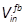, resulting from the combined action of the input and feedback voltages, is:.

The above equation can be better understood using the equivalent circuit in Figure 13, as we already did in Section 3.4. In the circuit, power is supplied by the AC power supply, replacing the vacuum tube. Voltage drops, through the resistors in the circuit, proceeding clockwise. Suppose highest voltage is at the top of the AC power supply (the anode of the vacuum tube), and lowest voltage is at the bottom of the AC power supply (the cathode of the vacuum tube). Vin is the voltage difference between the input in and the cathode resistor end, opposite to the voltage source (opposite to the cathode). The voltage difference between the input in and the other cathode resistor end (between the grid and the cathode) is higher than Vin, because of the voltage drop introduced by Rk. Given that the voltage drop is Vfb,will be equal to Vin plus Vfb.

The AC power supply produces a voltage equal to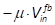. As we said in Section 3.4, the minus sign here indicates that the phase of the AC power supply is reversed with respect to that of. The output voltage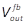is taken between the two ends of the load RL and can be computed using the voltage divider equation as.Figure 13: Equivalent circuit of the voltage amplifier with cathode resistorThe AC power supply produce a voltage equal to the amplification factor μ times the voltage between the input (grid of the vacuum tube) and the lower extreme of the voltage source itself. The minus sign indicates that the phase is reversed, with respect to the input signal. Each resistor introduces a voltage drop along the circuit, in the direction of the red arrow. The output signal is taken at the extremes of the load resistor RL. To neutralize the local negative feedback, a capacitor Ckcan be connected in parallel to Rk.

Using the voltage divider equation again, the feedback voltage Vfb is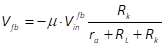.

Replacing Vfb into the equation forand simplifying we have:.

Now we can replace this into the equation forand we obtain:.

Finally, we can express the gain of the voltage amplifier, with local feedback introduced by a non-bypassed cathode resistor, as: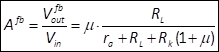.

As we said in Section 3.4, we do not consider the phase so we omit the minus sign.

Let us now consider the case where a capacitor Ck is used to bypass the cathode resistor Rk, as shown in Figure 13. The impedance of the capacitor depends on the signal frequency and is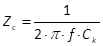.

The impedance of the cathode resistor in parallel with the bypass capacitor is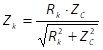.

The gain of the voltage amplifier, with local feedback introduced by a cathode resistor and bypassed by a capacitor, is obtained by replacing Rk with Zk in the equation for Afb. We obtain:.

The value of the gaindepends on the frequency and on the capacitor. It ranges between these two extremes:.

Minimum gain, equal to the non-bypassed cathode resistor, occurs when the capacitor impedance is maximum (at very low frequencies and/or very low capacitances). Maximum gain, similar to the circuit without cathode resistor, occurs when the capacitor impedance is minimum (at high frequencies and/or large capacitance).

The value of the capacitor should be chosen so that gain is maximum even at very low audible frequencies, as discussed in next example.

 Example 5: Gain of a voltage amplifier with self-bias Let us consider again the configuration of a voltage amplifier with the green loadline in Figure 8. In Example 1, we set the load RL to 150K Ohm. In Example 2 we determined that the anode resistance ra is 75K Ohm, and the gain of the amplifier at the operating point identified by the red spot, with no cathode resistor, is 66.6. In Example 4, we determined that the cathode resistor needed to set the operating point at the red spot, using self-bias, is 2K Ohm. Remember that he amplification factor of a 12AX7 vacuum tube is μ=100. Therefore, the gain of the voltage amplifier, using self-bias with this cathode resistor is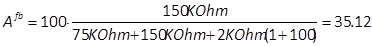. Considering that the full gain with no cathode resistor is 66.6, the new gain corresponds to 20∙log(35.12/66.6) = -5.5dB with respect to full gain. Suppose now we use a bypass capacitor of 150μF. At a frequency of f=1 Hz the capacitor impedance is. The impedance of the cathode resistor in parallel with the bypass capacitor is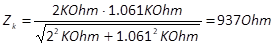. The gain of the voltage amplifier at 1 Hz, with the bypass capacitor, is:. This corresponds to 20∙log(47/66.6) = -3dB with respect to full gain. Repeating the same process for f=10Hz, we obtain a gain of 63.6, corresponding to 20∙log(63.6/66.6) = -0.4dB. This is fairly acceptable, since no attenuation is realistically perceived at all audible frequencies. Figure 14 reports the output gain attenuation, with the above configuration, with frequency ranging up to 100 Hz. It can be seen that the attenuation is practically eliminated from 40Hz on. Figure 14: Output gain attenuation with self-bias and bypass capacitor. Using a capacitor of 150μF we have -3dB output gain attenuation at 1 Hz and -0.4dB at 10 Hz. Attenuation is practically eliminated at 40 Hz.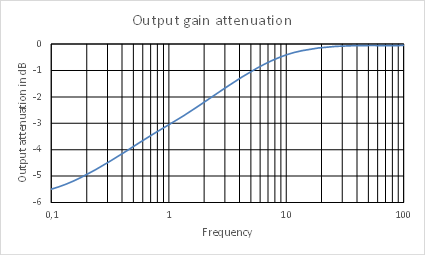Figure 14: Output gain attenuation with self-bias and bypass capacitor.Using a capacitor of 150μF we have -3dB output gain attenuation at 1 Hz and -0.4dB at 10 Hz. Attenuation is practically eliminated at 40 Hz.